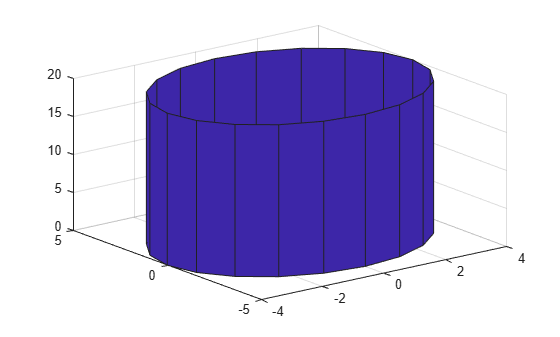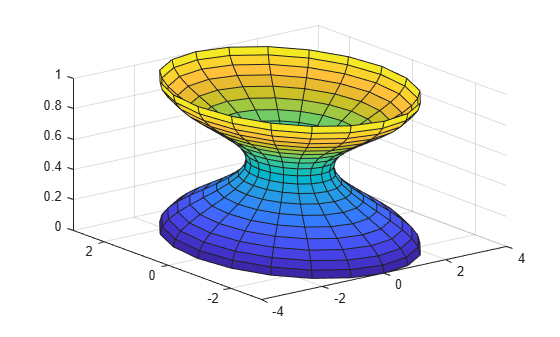Main Content

# cylinder

Create cylinder

## Syntax

``[X,Y,Z] = cylinder``
``[X,Y,Z] = cylinder(r)``
``[X,Y,Z] = cylinder(r,n)``
``cylinder(___)``
``cylinder(ax,___)``

## Description

example

````[X,Y,Z] = cylinder` returns three `2`-by-`21` matrices containing the x-, y-, and z- coordinates of a cylinder without drawing it. The cylinder has a radius of 1 and 20 equally spaced points around its circumference. The bases are parallel to the xy-plane.To draw the cylinder, pass `X`, `Y`, and `Z` to the `surf` or `mesh` function.```

example

````[X,Y,Z] = cylinder(r)` returns the x-, y-, and z- coordinates of a cylinder with the specified profile curve, `r`, and 20 equally spaced points around its circumference. The function treats each element in `r` as a radius at equally spaced heights along the unit height of the cylinder. The size of each coordinate matrix is `m`-by-`21`, where `m=numel(r)`. However, if `r` is a scalar, then `m=2`.```
````[X,Y,Z] = cylinder(r,n)` returns the x-, y-, and z- coordinates of a cylinder with the specified profile curve, `r`, and `n` equally spaced points around its circumference. The size of each coordinate matrix is `m`-by-(`n+1`), where `m=numel(r)`. However, `r` is a scalar, then `m=2`.```

example

````cylinder(___)` plots the cylinder without returning the coordinates. Use this syntax with any of the input arguments in previous syntaxes.```
````cylinder(ax,___)` plots into the axes specified by `ax` instead of the current axes. Specify the axes as the first input argument.```

## Examples

collapse all

Create and plot a cylinder with a radius equal to 1.

`cylinder`Specify the radius of a cylinder by including the input `r`. Then, specify the height of the cylinder by modifying the returned `Z` coordinate.

Define `X`, `Y`, and `Z` as coordinates of a cylinder with a radius of 4.

```r = 4; [X,Y,Z] = cylinder(r);```

Specify a height of 20 by modifying the `Z` coordinate. Plot the cylinder.

```h = 20; Z = Z*h; surf(X,Y,Z)```Create a cylinder and use the returned coordinates to plot multiple cylinders in different locations.

Create a cylinder defined by the profile function `2 + cos(t)`.

```t = 0:pi/10:2*pi; r = 2 + cos(t); [X,Y,Z] = cylinder(r);```

Plot the cylinder with the base centered at the origin.

`surf(X,Y,Z)`Plot two more cylinders on top of the first cylinder.

```hold on surf(X,Y,Z+1) surf(X,Y,Z+2)```## Input Arguments

collapse all

Profile curve, specified as a vector. `cylinder` treats each element in `r` as a radius at equally spaced heights along the unit height of the cylinder.

Number of points around the cylinder circumference, specified as a positive whole number.

Target axes, specified as an `Axes` object. If you do not specify the axes, then `cylinder` plots into the current axes.

## See Also

Introduced before R2006a

Download ebook